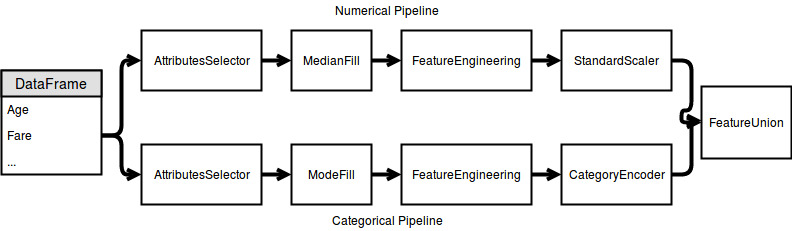# Pipeline with Scikit

by Daniel Pollithy — on  ,  ,

## Processing data with scikit’s pipelines, pandas and numpy

Fiddling around with my first kernels on Kaggle I found processing data time consuming and it easily looked messy in the jupyter notebook. Especially the fact that I splitted the data set and therefore had to wrap every transformation into a method which I could call on the parts was annoying.

But the solution was always there: Pipelines.

## Designing a pipelineI am going to show you the code snippets which I used to achieve 80% accuracy on the Titanic test data.

Inspired by “Hands on Machine Learning with Scikit-Learn and TensorFlow” I split the pipeline into two single pipelines. One for the categorical and the other for the numerical attributes.

``````# Name is part of the numerical attributes because I want to use it for feature engineering
num_attribs = [u'Pclass', u'Age', u'SibSp', u'Parch', u'Fare', u'Name']
cat_attribs = [u'Sex', u'Embarked', u'Name']
``````

## Writing an estimator/transformer

Scikit uses Duck-Typing which follows the rule:

If it looks like a duck and quacks like a duck, it’s a duck.

So we don’t have to use inheritance but implement the necessary methods to be counted as a duck (in our case an estimator). But to unlock the power of grid search I still used inheritance.

``````class UselessStepInThePipeline(BaseEstimator, TransformerMixin):
def __init__(self):
pass
def fit(self, X, y=None):
return self
def transform(self, X, y=None):
return X
``````

## Writing the necessary steps

As you can see in the image: The data is piped through some nodes. Except for the StandardScaler and the FeatureUnion all of them have to be written.

Because pandas is so convenient I took care to not convert pandas DataFrame to a ndarray which happens in the “Hands on Machine Learning …” book.

### The AttributeSelector

This transformer copies the input matrix and drops all features which are not selected.

``````class DataFrameSelector(BaseEstimator, TransformerMixin):
def __init__(self, attribute_names):
self.attribute_names = attribute_names
def fit(self, X, y=None):
return self
def transform(self, X):
for column_name in X.columns:
if column_name not in self.attribute_names:
X = X.drop([column_name], axis=1)
return X
``````

### The Fill Transformer

Empy cells are loaded with NaN by pandas. We can’t pass NaNs to the models we want to train so we have to either drop sparse features or fill them with values.

In my first try on the Titanic dataset I filled numerical attributes with the Median and categorical attributes with the Mode.

The following estimators take care of this (although they could be merged into one):

``````class PandasMedianFill(BaseEstimator, TransformerMixin):
def __init__(self, columns=None):
self.columns = columns
pass
def fit(self, X, y=None):
return self
def transform(self, X, y=None):
columns = self.columns if self.columns is not None else X.columns
for column in columns:
X[column].fillna(X[column].median(), inplace = True)
return X

class PandasModeFill(BaseEstimator, TransformerMixin):
def __init__(self, columns=None):
self.columns = columns
pass
def fit(self, X, y=None):
return self
def transform(self, X, y=None):
columns = self.columns if self.columns is not None else X.columns
for column in columns:
X[column].fillna(X[column].mode(), inplace=True)
return X
``````

### Feature Engineering

The numerical pipeline will be enriched by the length of the name of the person:

``````class NameLengthAdder(BaseEstimator, TransformerMixin):
def __init__(self): # no *args or **kargs
pass

def fit(self, X, y=None):
return self  # nothing else to do

def transform(self, X, y=None):
X['NameLength'] = X['Name'].str.len()
X = X.drop(['Name'], axis=1)
return X
``````

The categorical pipelines obtains the new feature Title.

``````class CatAttributesAdder(BaseEstimator, TransformerMixin):
def __init__(self, stat_min=10): # no *args or **kargs
self.stat_min = stat_min

def fit(self, X, y=None):
return self  # nothing else to do

def transform(self, X, y=None):
# create new features
X['Title'] = X['Name'].str.split(", ", expand=True).str.split(".", expand=True)
title_names = (X['Title'].value_counts() < self.stat_min)
X['Title'] = X['Title'].apply(lambda x: 'Misc' if title_names.loc[x] == True else x)

X = X.drop(['Name'], axis=1)

return X
``````

### Last step

The categorical data like the Title has to be decomposed into single boolean attributes. For example for every possible string a new attribute. This method is called “one-hot” encoding. Be aware that the training and test data don’t have to present the same set of possible values. In that case the following method would not work correctly:

``````Definition of the CategoricalEncoder class, copied from PR #9151.
CategoricalEncoder(encoding="onehot-dense")
``````

(The dense means that a real numpy array is used and not a scipy sparse matrix.)

Numerical attributes have to be scaled because if not attributes with large values would skew every model we would like to fit. We use the builtin StandardScaler.

## Putting everything together

The beauty of the pipelines lies in the neat way of writing them.

``````num_pipeline = Pipeline([
('selector1', DataFrameSelector(num_attribs)),
('pandas_median_fill', PandasMedianFill()),
('std_scaler', StandardScaler()),
])

cat_pipeline = Pipeline([
('selector2', DataFrameSelector(cat_attribs)),
('pandas_mode_fill', PandasModeFill()),
('cat_encoder', CategoricalEncoder(encoding="onehot-dense")),
])

full_pipeline = FeatureUnion(transformer_list=[
("num_pipeline", num_pipeline),
("cat_pipeline", cat_pipeline),
])
``````

Now using them again and again is as easy as writing the following code:

``````data_train = pd.read_csv('../input/train.csv')
train = data_train.copy()
train_labels = data_train['Survived'].copy()
train = full_pipeline.fit_transform(data_train)
``````

Pouring them into a model looks like we never had any categorical data or strings containing unwanted attributes:

``````# Learn a RandomForest
from sklearn.ensemble import RandomForestClassifier
from sklearn.model_selection import cross_val_score

forest_reg = RandomForestClassifier(random_state=42)
forest_reg.fit(train, train_labels)

train_predictions = forest_reg.predict(train)

# how many hits/total do we have?
train_score = accuracy_score(train_labels, train_predictions)
print("Train score: {}".format(train_score))
``````